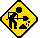## Sharp EL-5120

Datasheet legend
Ab/c: Fractions calculation
AC: Alternating current
BaseN: Number base calculations
Card: Magnetic card storage
Cmem: Continuous memory
Cond: Conditional execution
Const: Scientific constants
Cplx: Complex number arithmetic
DC: Direct current
Eqlib: Equation library
Exp: Exponential/logarithmic functions
Fin: Financial functions
Grph: Graphing capability
Hyp: Hyperbolic functions
Intg: Numerical integration
Jump: Unconditional jump (GOTO)
Lbl: Program labels
LCD: Liquid Crystal Display
LED: Light-Emitting Diode
Li-ion: Lithium-ion rechargeable battery
Lreg: Linear regression (2-variable statistics)
mA: Milliamperes of current
Mtrx: Matrix support
NiMH: Nickel-metal-hydrite rechargeable battery
Prnt: Printer
RTC: Real-time clock
Sdev: Standard deviation (1-variable statistics)
Solv: Equation solver
Subr: Subroutine call capability
Symb: Symbolic computing
Tape: Magnetic tape storage
Trig: Trigonometric functions
Units: Unit conversions
VAC: Volts AC
VDC: Volts DC
 Years of production: Display type: Alphanumeric display New price: Display color: Black Display technology: Liquid crystal display Size: 6"×3"×½" Display size: characters Weight: 4 oz Entry method: Formula entry Batteries: 2×"LR44" button cell Advanced functions: Trig Exp Hyp Lreg Cmem External power: Memory functions: +/- I/O: Programming model: Formula programming Precision: 12 digits Program functions: Jump Cond Subr Lbl Memories: 1211(0) bytes Program display: Formula display Program memory: 1211 bytes Program editing: Formula entry Chipset: Forensic result: 8.99998762105The EL-5120 is a formula programmable scientific calculator.```INPUT X
Y=1
LABEL 1
IF X>5 GOTO 2
Y=Y*X
X=X+1
GOTO 1
LABEL 2
G=(X*ln X-X+ln (2*π/X)/2+(((1/1260-1/1680/X/X)/X/X-1/360)/X/X+1/12)/X
e^G/Y```